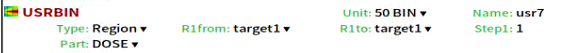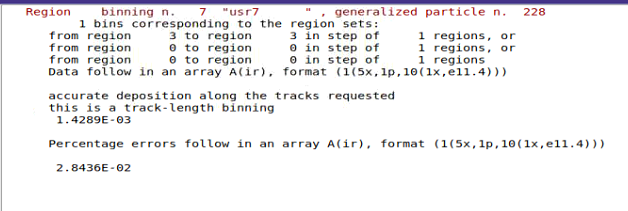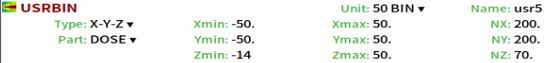# Normalization of USRBIN DOSE results

Furthermore,I’am also intersted in the absorbed dose by target1 in my case. Thus,I change the requested quantity to dose and get the results:According to your reply,here’s my assmuption to obtain the total absorbed dose by target1 under the condition of 1mA electron accelerator for ten seconds:

As the result of USRBIN is in units of GeV/g, firstly I should multiply it by 1.602E-7 to obtain dose in Gy; and then by 6.2415E15( primary particles per second ) and 10 (duration).

Therefore,we have the following equation to calculate the absorbed dose by target1 in ten seconds:
1.4289E-03 * 1.602E-7 * 6.241E15 * 10= 1.4286E7 (Gy)

As a beginer, I’d like to check whether there is anything wrong with my assumption. I would appreciate it if you could help to check my assumption.

Kind regards,
shiqiang pang

1 Like

Dear @shiqiang.pang ,

As @amario said, your reasoning seems correct. However, there is a problem with your initial assumption: the units of the result provided by FLUKA in this case are GeV*cm^3/g, so you should divide it by the volume of your region ( in cm^3 ) before you can get the result in GeV/g and proceed as detailed in your post.

Note that, in general, FLUKA does not know the volume of your region so it cannot obtain its mass and therefore will not provide the result in GeV/g when USRBIN type Region is requested.

Kind regards,
Francisco

Dear @fogallar,

Volumes of region are not mentioned here.

It seems that the dose plot is in units of GeV/g (per primary)DOSE----type Region: in units of GeV*cm^3/g (per primary)
DOSE----type XYZ(plot): in units of GeV/g (per primary)

By the way,what’s situlation when “NET-CHRG” is selected?
NET-CHRG----type Region: in units of elementary charge (per primary)
NET-CHRG----type XYZ(plot): in units of :

Dear @shiqiang.pang,

The manual also states in Note 13:

The results from USRBIN are normalised per unit volume and per unit primary weight, except region binnings and special user-defined binnings, which are normalised per unit primary weight only, […]

So the units you specified are correct, the unit of NET-CHRG in a Cartesian binning is Charge/cm^3.

Cheers,
David

Dear @horvathd,
So here comes the diffrence between the normalization of DOSE and NET-CHRG when type Region is selected. For DOSE normalization, volume of target1 is taken into account, while NET-CHRG is not. Is it correct?

Dear @shiqiang.pang,

the manual is correct, as the Cartesian (Cylindrical, etc.) scoring is always normalized with the volume of the bin, while the Region scoring not.

The reason for this is, that FLUKA can calculate the volume of a bin, but not of a region.

The difference you see in the Dose scoring, is because how Dose is calculated:
Fluka scores the deposited energy divided by the density of the material, so the unit is: GeV \cdot cm^3 / g.

In case of a Cartesian scoring, this is further divided by the volume of the bin as well, making the unit: GeV / g.

Cheers,
David

1 Like

Thanks for the explanation.Now I understand.

Kind regards,
shiqiang pang